Courses
Courses for Kids
Free study material
Free LIVE classes
More

# Long Division MethodLIVE
Join Vedantu’s FREE Mastercalss

## Introduction to Long Division Problems

The division is one of the four basic operations of arithmetic, the ways that numbers are combined to make new numbers. The other operations are addition, subtraction, and multiplication.

Long division is one method in which the concept of division, multiplication, and subtraction is used simultaneously.

Not only is this about division, but students must also understand the use of long division, and the meaning of divisors, dividends, and multiples.

In Mathematics, long division problems are the mathematical method for dividing large numbers into smaller groups or parts. It helps to break down a problem into simple and easy steps.

For example, 4 people having pizza now using this application can be divided up into 8 equal parts. Now with this, each person gets 2 slices.Dividing pizza among 4 people.

Now with this, each person gets 2 slices.

These symbols are used in different forms for example:

• x ÷ y

• x/y

In this article, long division worksheets and long division problems have been given.

## Elements of Division

Here’s one by one element of long division with the following examples.

• Dividend- The dividend is the number you are dividing up with

• Divisor- The divisor is the number you are dividing by

• Quotient-  It is the answer

• The Remainder- If the answer to the division problem is not a whole number the rest of the number is called the remainder.

Therefore,

Dividend ÷ Divisor = Quotient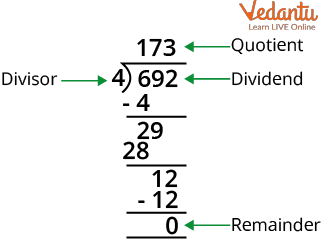Elements of the Division

## Steps to Carry Out in Long Division

We will carry out 5 steps to solve every long division with ease.

• Divide

• Multiply

• Subtract

• Bring down

• RemainderSteps Involved in Long Division

## Properties of Long Division

• Division by 1 Property: If we divide a number by 1 the quotient is the number itself. Or in other words, when any number is divided by 1, we always get the number itself as the answer/quotient.

For Example:

(i) 7592 ÷ 1 = 7592

(ii) 5247 ÷ 1= 5247

• Division by the Same Number Property: If we divide a number by the number itself then the quotient is always 1.

For Example:

(i) 275 ÷ 275 = 1
(ii) 105 ÷ 105 = 1

• Division of Any Number by 0 Property is meaningless

For Example:

(i) 35 ÷ 0 = no meaning

(ii) 65 ÷ 0 = no meaning

• Division of 0 by Any Number Property: If 0 is divided by a number gives 0 as the quotient or answer. In other words, when 0 is divided by any number, we always get 0 as an answer.

For example:

(i) 0 ÷ 25 = 0

(ii) 0 ÷ 100 = 0

## Solved Example of Long Division Worksheet

Example 1: Below is the worksheet to solve the questions by long division methods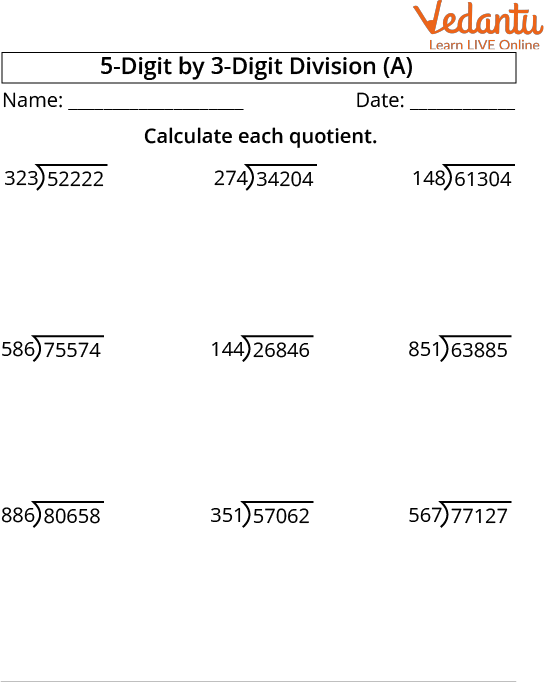Question on Long Division

Ans: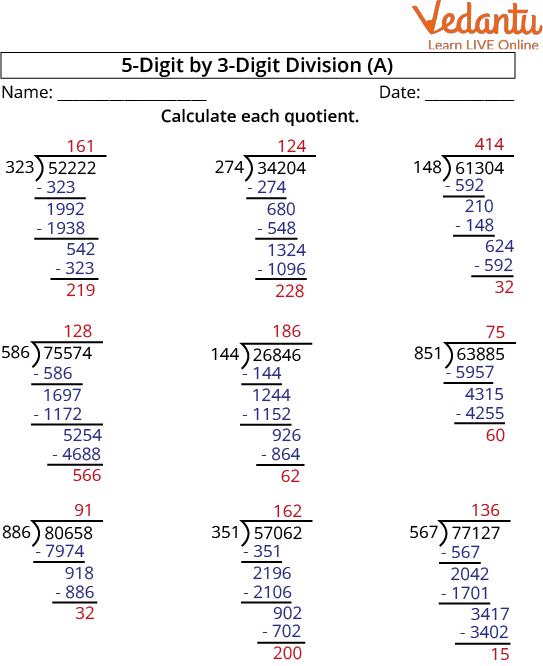Solution of long division

Example 2: Find the value of quotient and remainder when 75 is divided by 3 ? Verify using the Long division method.

Ans: Here, we divided 75 by 3. So, the dividend is 75 and the divisor is 3.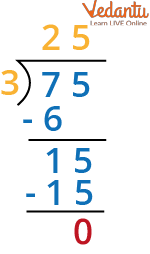Division of 75 by 3

Hence, we get the quotient as 25 & remainder as 0.

To check division we will put the value.

Dividend = (Divisor × Quotient) + Remainder.

Therefore, 75 = 3 × 25 + 0 = 75

Example 3. Divide 9.24 by 7

Ans: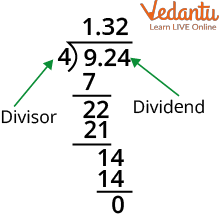Division with decimal

## Practice Problem Related to Long Division

Q1. Divide the 852.8 ÷ 6 and give the exact answer.

Ans: 142.133

Q2. Find the quotient when 7859 is divisible by 76.

Ans: 103.4

Q3. Find the quotient when 91 is divided by 9 using the long division method.

Ans: 10.11

Q4. Divide 324 by 2.

Ans. 162

## Summary

All above it is described that long division is a separation of something into different parts. It is a tool that helps to divide things into different parts and it includes elements such as Dividend, Divisor, Quotient, and Remainder. We dealt with the different properties of division and some solutions with solved examples.

Last updated date: 22nd Sep 2023
Total views: 119.4k
Views today: 1.19k

## FAQs on Long Division Method

1. Why is Division by zero undefined?

Division by zero is not defined because we cannot divide any number by 0. This is because when any number is multiplied by 0 the answer is also 0. Now if we do the opposite of it like 1 is divided by 0 then we will get the infinite value which is not quantifiable in mathematics.

2. What is the division symbol and what are some strategies for long division?

There are two signs of long division which are ÷ & /

The steps are more or less the same, except for new addition:

• Divide the tens column dividend by divisor.

• Multiply the divisor by the quotient in the tens place column.

• Subtract the product from the divisor.

• Bring down the dividend in one column and repeat.

3. Mention the divisibility rules of 6 and 8.

The rules of divisibility of 6 and 8 are:

• Divisibility by 6: Numbers divisible by 6 can also be divided by both 3 and 2. Students should test the number with both rules for 3 and 2. If the number passes both tests, it can be divided by 6. If it fails just one test it cannot.

For instance:

308 ends in an even digit, so it’s divisible by 2. However, 3 + 0 + 8 = 11, which cannot be divided evenly by 3. As such, 308 is not divisible by 6.